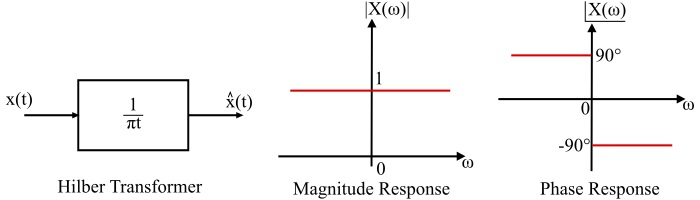# Signals & Systems â€“ What is Hilbert Transform?

## Hilbert Transform

When the phase angles of all the positive frequency spectral components of a signal are shifted by (-90°) and the phase angles of all the negative frequency spectral components are shifted by (+90°), then the resulting function of time is known as Hilbert transform of the given signal.

In case of Hilbert transformation of a signal, the magnitude spectrum of the signal does not change, only phase spectrum of the signal is changed. Also, Hilbert transform of a signal does not change the domain of the signal.

Let a signal x(t) with Fourier transform X(ω). The Hilbert transform of x(t) is obtained by the convolution of x(t) and (1/πt), i.e.,

$$\mathrm{\hat{x}(t)=x(t)*(\frac{1}{\Pi\:t})}$$

$$\mathrm{\Longrightarrow\hat{x}(t)=\frac{1}{\pi}\int_{-\infty}^{\infty}\frac{x(\tau)}{t-\tau}d\tau}$$

Also,

$$\mathrm{\hat{x}(t)=\frac{1}{\pi}\int_{-\infty}^{\infty}\frac{x(t-\tau)}{\tau}d\tau}$$

It shows that the Hilbert transform of the signal x(t) is a linear operation. This definition of the Hilbert transform is applicable to all the signals which are Fourier transformable.

## Inverse Hilbert Transform

The process of recovering the original signal x(t) from $\hat{x}(t)$ is called the inverse Hilbert transform. Mathematically, it is defined as,

$$\mathrm{x(t)=-\frac{1}{\pi}\int_{-\infty}^{\infty}\frac{\hat{x}(\tau)}{t-\tau}d\tau}$$

The equations of functions x(t) and $\hat{x}(t)$together are called Hilbert transform pair.

## Explanation

As the Hilbert transform $\hat{x}(t)$ of a signal x(t) obtained by the convolution of x(t) with (1/πt). Therefore, from the convolution property of Fourier transform, the convolution of two time domain functions can be transformed into the multiplication of their Fourier transform in the frequency domain.

The Fourier transform of signal (1/πt) is defined as,

$$\mathrm{F=\left[\frac{1}{\pi\:t}\right]=-j\:sgn(\omega)}$$

Where, sgn(ω) is the signum function in the frequency domain and is defined as

$$\mathrm{sgn(\omega)=\left\{\begin{matrix} 1;\: \omega >0 \ -1;\: \omega <0 \ \end{matrix}\right.}$$

Now, the Fourier transform of $\hat{X}(ω)$ of $\hat{x}(t)$ is given by,

$$\mathrm{F[\hat{x}(t)]=\hat{X}(\omega)=-j\:sgn(\omega).X(\omega)}$$

It means,

$$\mathrm{\hat{X}(\omega)=\left\{\begin{matrix} -jX(\omega);\:\:\omega>0 \ jX(\omega) ;\:\:\omega<0 \end{matrix}\right.}$$

This expression states that the Hilbert transform $\hat{x}(t)$ of a signal x(t) may be obtained by passing x(t)through a linear device whose transfer function is $[-j\:sgn(\omega)]$. This linear device may be considered as the one that produces a phase shift of (-90°) for all positive frequencies of the input signal and (+90°) for all the negative frequencies.

Also, the magnitudes of all the frequency spectral components in the signal are unaffected by the transmission of the signal through the device, i.e.,

$$\mathrm{\left|\hat{X}(\omega)\right |=\left |X(\omega)\right|}$$

Such an ideal device is known as Hilbert transformer. The Hilbert transformer may be viewed as an ideal all pass 90° phase-shifter. The Hilbert transformer is not causal i.e. it is not physically realizable.

The graphical representation of Hilbert transformer with its magnitude response and phase response is shown in the figure.Important − The Hilbert transform of the product of a low-pass signal and a high-pass signal is equal to the product of the low-pass signal and the Hilbert transform of the high-pass signal. Therefore, if x(t) is a low-pass signal and y(t) is a high-pass signal, then,

$$\mathrm{\widehat{x(t).y(t)}=x(t).\widehat{y(t)}}$$

## Applications of Hilbert Transform

The Hilbert transform is mainly used in the field of signal processing, analysis and synthesis of signals and design of filters, etc. Some chief applications of the Hilbert transform are given as −

• Hilbert transform is used to represent the band pass signals.

• Hilbert transform is used to realise the phase selectivity in the generation of single-sided band (SSB) modulation system.

• The Hilbert transform is also used to relate the gain and phase characteristics of the linear communication channels and the minimum phase type filters.

## Numerical Example

Find the Hilbert transform of signal given as,

$$\mathrm{x(t)=sin\:\omega_{0}t}$$

Solution

The given signal is,

$$\mathrm{x(t)=sin\:\omega_{0}t}$$

From the definition of Fourier transform, we have,

$$\mathrm{F[sin\omega_{0}t]=X(\omega)=-j\pi[\delta(\omega-\omega_{0})-\delta(\omega+\omega_{0})]}$$

Also, the Fourier transform of the Hilbert transform of x(t) is

$$\mathrm{F[\hat{x}(t)]=\hat{X}(\omega)=-j\:sgn(\omega).X(\omega)}$$

$$\mathrm{\therefore\hat{X}(\omega)=\left \{ -j\:sgn(\omega) \right \}\left \{-j\pi [\delta (\omega -\omega_{0})-\delta (\omega +\omega_{0})] \right \}}$$

$$\mathrm{\Longrightarrow\hat{X}(\omega)=-\pi [\delta (\omega -\omega_{0})-\delta (\omega +\omega_{0})]sgn(\omega)}$$

$$\mathrm{\Longrightarrow\hat{X}(\omega)=-\pi [\delta (\omega -\omega_{0})+\delta (\omega +\omega_{0})]}$$

Therefore, the Hilbert transform of the given signal is

$$\mathrm{\hat{x}(t)=F^{-1}\left \{-\pi[\delta(\omega-\omega_{0})+\delta(\omega+\omega_{0})] \right \}=-cos\omega_{0}t}$$

Or, it can also be represented as

$$\mathrm{\widehat{sin\omega_{0}t}=-cos\omega_{0}t}$$